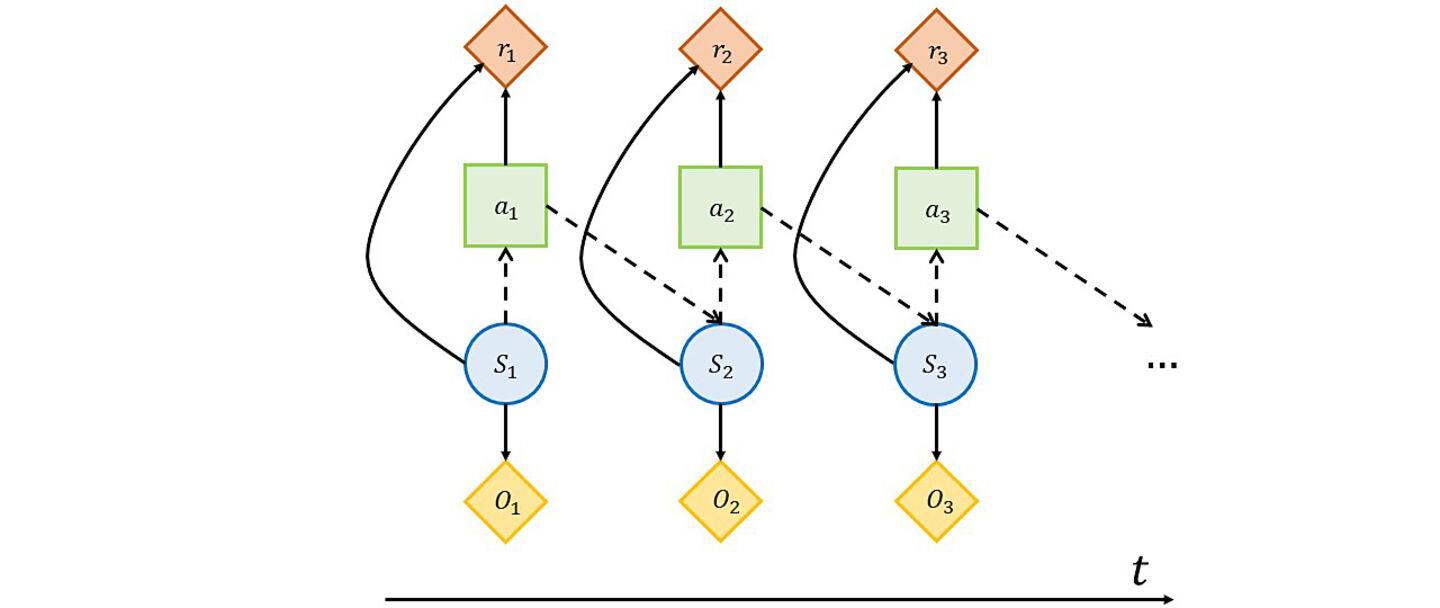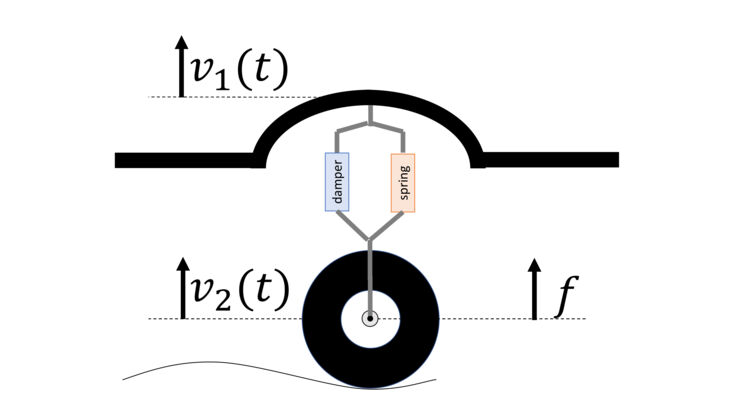# Reinforcement Learning for Partially Observable Dynamical systemsA partially observable dynamical system is shown in the figure. $S_t$ represents the state variable which is not measurable and instead, the output $O_t$ is measurable. Our aim is to use $O_k$ for $k \leq t$ to design the action $a_t$ such that the future rewards $r_k$ for $k >t$ are maximized. A central issue in this problem is that dynamics (shown by dashed lines) is unknown. An illustrative example is given a few lines below.

This project aims at developing end-to-end model-free Reinforcement Learning (RL) algorithms, for control of partially observable dynamical systems with continuous state and control spaces.

## Introduction to Reinforcement Learning

Machine Learning (ML) has surpassed human performance in many challenging tasks like playing video games and classification. By recent progress in ML, specifically using deep networks, there is a renewed interest in applying ML techniques to control autonomous systems interacting with a physical environment. There are many opportunities in developing data-driven algorithms for control of autonomous systems to do interactive tasks like autonomous driving, agile robotics, etc.

Adaptive control studies data-driven approaches for control of unknown dynamical systems. Combining with optimal control theory it is possible to control unknown systems adaptively and optimally. Reinforcement Learning (RL) refers to a class of such routines and its history dates back decades. By recent progress in ML, specifically using deep networks, the RL field is also reinvented. RL algorithms have recently shown impressive performances in many challenging problems including playing Atari games, agile robotics, control of continuous-time systems, and distributed control of multi-agent systems.

## Reinforcement Learning for Partially Observable Dynamical Systems

Reinforcement Learning aims to provide an end-to-end framework to control a dynamic environment. The input to the RL framework is raw sensory data and the output is an action which, in the end, will converge to the optimal action. The RL framework might be a simple single-layer or a very complicated and deep neural network which is trained to learn the optimal action. This end-to-end framework seems interesting but in some cases, it fails to achieve the objective independent of how well we have trained the network. The reason is that the sensor data does not contain all information about the state variable to put it simply. This motivates the study of RL for partially observable dynamical systems.

## Project Description

This project aims at developing end-to-end model-free RL algorithms for control of partially observable dynamical systems with continuous state and control spaces. We consider dynamical systems whose state- and action-space are continuous. We assume that not all states are observable and the system is only partially observable. Using partial observations, we aim to develop end-to-end model-free RL algorithms to control the system to perform a given task. Note that the given task may change from one to another and it is desired that the RL algorithm learns to control the system to perform the new task properly without being tuned by humans.

## An illustrative example

Consider a dynamical system of a car mounted on a tire. Let v1 denote the car vertical velocity which is measured, v2 denote the wheel vertical velocity which is not measured and f denote the input to the system; i.e. a force that can be applied to the rim center.Photo credit: Farnaz Adib Yaghmaie

The control objective is defined as detecting a loose wheel and compensating its effect by designing f. To detect a loos wheel and compensate its effect, one might need to know the wheel vertical velocity v2 which is not directly observable. There are many challenges in addressing this problem. A few of them is listed below:

• The first step in solving this control problem is to estimate v2 which is not directly observable. This requires modeling the dynamical system which usually needs much effort and contains many simplifying assumptions. The better model, the better observer design.
• To compensate for the effect of the loose wheel, one might need to minimize a cost function. The cost function is designed to result in the desired behavior and might be quite complicated. So, it is required to solve an optimal control problem where the dynamics is nonlinear and imprecise, and the cost function is, in general, non-quadratic.
• If the dynamics of the system changes abruptly, the previously obtained optimal control is not valid anymore and might not satisfy the basic control requirements.

In this project, we aim to develop end-to-end model-free RL algorithms to control unknown dynamical system to perform a desired task. The main features of such algorithms are:

• There is no need to model the dynamics. Instead of learning the dynamics, the RL algorithm directly learns the optimal control from sensory data. In this sense, the RL algorithm provides an end-to-end framework from sensory observation v1 to control input f. If the model of the system changes or some external signals disturb the system, the RL algorithm modifies the solution automatically.
• There is no need to design an observer and tune its parameter.
• The RL algorithm can reason about unpredicted conditions based on previous experiences.

Mathematically speaking, we aim to develop end-to-end model-free RL algorithms for control of partially observable dynamical systems with continuous state and control spaces (states and control can change over some intervals so they are continuous).

• This project aims at developing end-to-end model-free Reinforcement Learning (RL) algorithms, for control of partially observable dynamical systems with continuous state and control spaces.
• The expected duration of the project is six years, 2021-2026.
• The research activities will be performed within the Division of Automatic Control, in collaboration with ABB AB research corporate, SAAB AB, and Nira Dynamics.
• This research is supported by Centrum för Industriell Informationsteknologi (CENIIT).